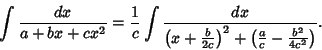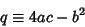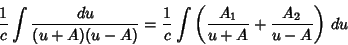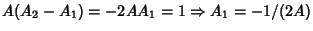To compute an integral of the form(1)

Complete the Square in the Denominator to obtain(2)

Let. Then define(3)

where(4)

is the Negative of the Discriminant. If, then(5)

Now use Partial Fraction Decomposition,(6)(7)

soand. Plugging these in,(8)
for. Note that this integral is also tabulated in Gradshteyn and Ryzhik (1979, equation 2.172), where it is given with a sign flipped.

References

Gradshteyn, I. S. and Ryzhik, I. M. Tables of Integrals, Series, and Products, 5th ed. San Diego, CA: Academic Press, 1979.